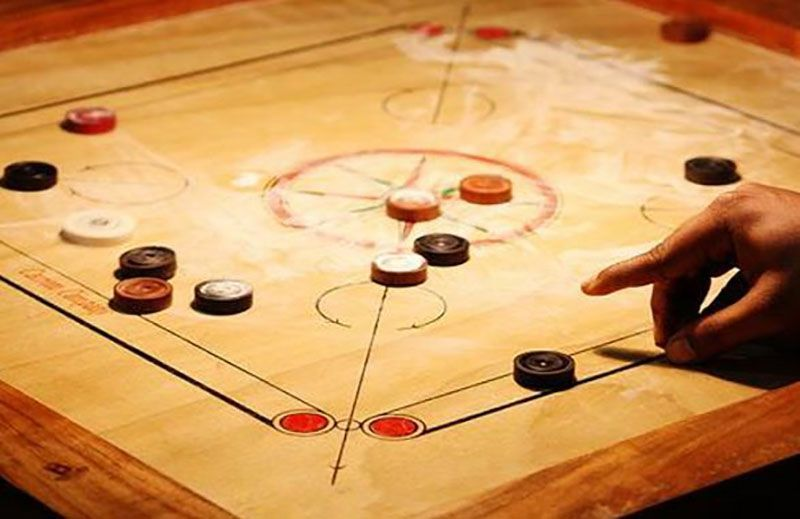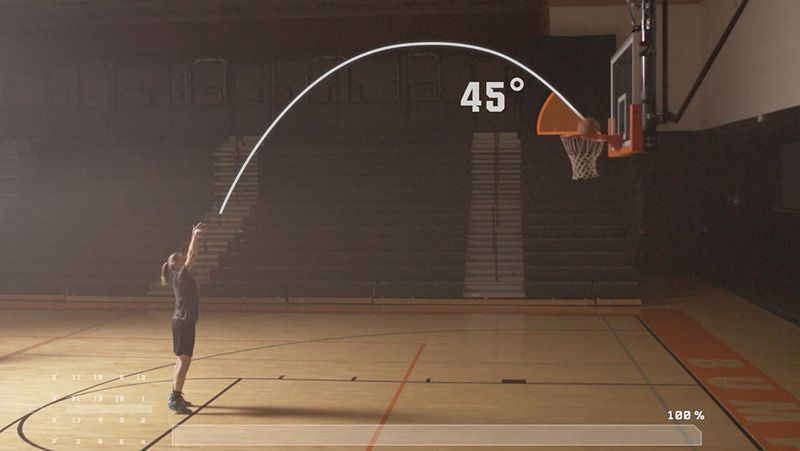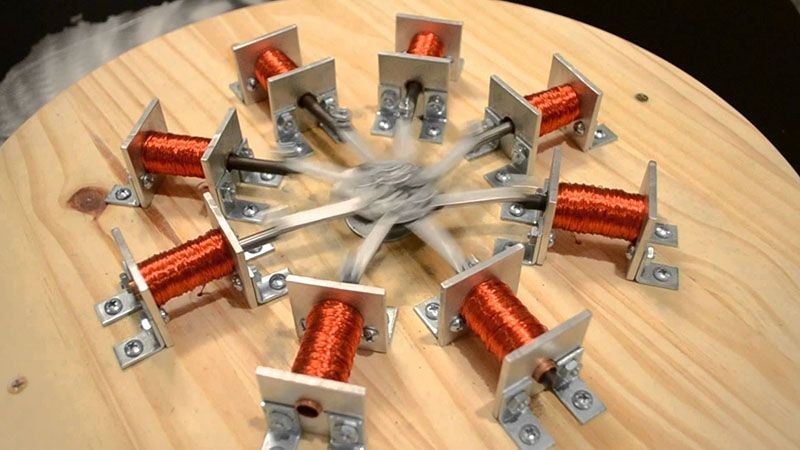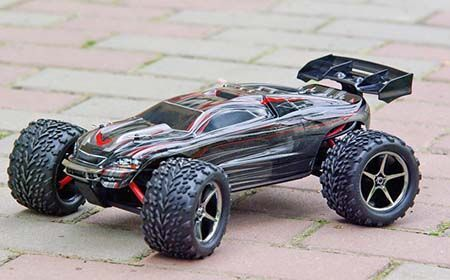# Motion

Motion occurs when an object changes its position.

Motion is the change in position of an object with respect to the observer.

# Translatory Motion

Translatory motion is that in which all the particles move through the same distance in the same time.

There are two main types of translatory mo-tion:

Rectillinear MotionIn rectilinear motion, the body moves in a straight line.

Curvillinear MotionIn curvilinear motion, the body moves along a curved line.

# Rotatory Motion

Rotatory motion is that in which a body moves about a fixed axis without changing its position.

# Oscillatory Motion

Oscillatory motion is a back and forth repetitive motion.

# Speed

Motion is a state of a moving body. The measure of motion (i.e., how fast/slow a ·body moves) is called speed.

# Uniform Motion

A body is said to have uniform motion when it travels equal distances in equal intervals of time. A body moving with uniform speed is said to be in uniform motion.

# Non-Uniform Motion

A body is said to have non-uniform motion when it travels unequal distances in equal intervals of time. A body moving with variable speed is said to be in non-uniform mo-tion.

# Uniform Motion

Marching is an exmaple of uniform motion where equal distances are covered in equal intervals of time.

# Non Uniform Motion

When a train halts at a station it covers lesser and lesser (different intervals of) distance in equal intervals of time.

# Access All Content

## More from Grade 8 Physics# Heating Effect of Electric Current

Understand the concept of heating effect of electric current. See real world examples where this effect is observed.# Magnetic Effect of Electric Current

Understand the concept of magnetic effect of electric current. Demystify this seemingly magical effect. See it's application in the real world.# Electric Circuit

Understand the basic components of a electrical circuit using an exciting video of RC car.